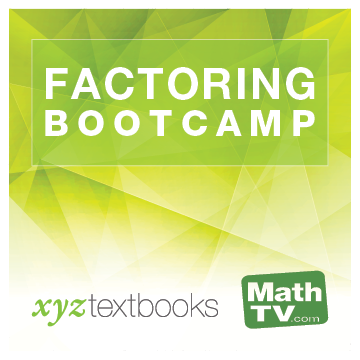#### Videos for:Factoring Bootcamp
Review: Sums, Differences, and Products of Polynomials

Select an Instructor to Play Video

Example 11

A company produces and sells copies of an accounting program for home computers. The total weekly cost (in dollars) to produce $$x$$ copies of the program is $$C(x)=8x+500$$. Find its weekly profit if the total revenue obtained from selling all $$x$$ programs is $$R(x)=35x-0.1x^2$$. How much profit will the company make if it produces and sells $$100$$ programs a week? That is, find $$P(100)$$.

Mini Lecture
Simplify.

1. $$2x^2-3x+10x-15$$

2. $$\left(5x^2+6x+1\right)-\left(4x^2+7x-2\right)$$

3. $$4x-5\left[3-(x-4)\right]$$

Mini Lecture
Multiply.

1. $$4a^3(3a^2-a+1)$$

2. $$(2a-3)(3a^2-5a+1)$$

3. $$(2a+3)(3a+2)$$

4. $$(x-2)^3$$Innovative products, peer tutors, fair prices, and unlimited access
www.xyztextbooks.com | info@mathtv.com